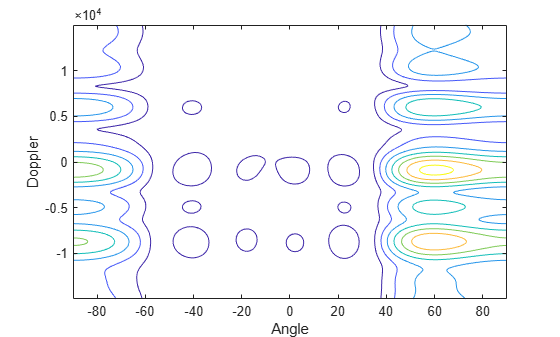# step

System object: phased.AngleDopplerResponse
Package: phased

Calculate angle-Doppler response

## Syntax

```[RESP,ANG_GRID,DOP_GRID] = step(H,X) [RESP,ANG_GRID,DOP_GRID] = step(H,X,ELANG) RESP,ANG_GRID,DOP_GRID = step(H,X,PRF) ```

## Description

Note

Starting in R2016b, instead of using the `step` method to perform the operation defined by the System object™, you can call the object with arguments, as if it were a function. For example, `y = step(obj,x)` and `y = obj(x)` perform equivalent operations.

```[RESP,ANG_GRID,DOP_GRID] = step(H,X)``` calculates the angle-Doppler response of the data `X`. `RESP` is the complex angle-Doppler response. `ANG_GRID` and `DOP_GRID` provide the angle samples and Doppler samples, respectively, at which the angle-Doppler response is evaluated. This syntax is available when the `ElevationAngleSource` property is `'Property'`.

The size of the first dimension of the input matrix can vary to simulate a changing signal length. A size change can occur, for example, in the case of a pulse waveform with variable pulse repetition frequency.

```[RESP,ANG_GRID,DOP_GRID] = step(H,X,ELANG)``` calculates the angle-Doppler response using the specified elevation angle `ELANG`. This syntax is available when the `ElevationAngleSource` property is `'Input port'`.

`RESP,ANG_GRID,DOP_GRID = step(H,X,PRF)` uses `PRF` as the pulse repetition frequency. This syntax is available when the `PRFSource` property is ```'Input port'```.

Note

The object performs an initialization the first time the object is executed. This initialization locks nontunable properties and input specifications, such as dimensions, complexity, and data type of the input data. If you change a nontunable property or an input specification, the System object issues an error. To change nontunable properties or inputs, you must first call the `release` method to unlock the object.

## Input Arguments

 `H` Angle-Doppler response object. `X` Input data as a matrix or column vector. If `X` is a matrix, the number of rows in the matrix must equal the number of elements of the array specified in the `SensorArray` property of `H`. If `X` is a vector, the number of rows must be an integer multiple of the number of elements of the array specified in the `SensorArray` property of `H`. In addition, the multiple must be at least 2. `ELANG` Elevation angle in degrees. You can specify this argument as single or double precision. Default: Value of `Elevation` property of `H` `PRF` Pulse repetition frequency specified as a positive scalar. To enable this argument, set the `PRFSource` property to ```'Input port'```. Units are in Hertz. You can specify this argument as single or double precision.

## Output Arguments

 `RESP` Complex angle-Doppler response of `X`. `RESP` is a P-by-Q matrix. P is determined by the `NumDopplerSamples` property of `H` and Q is determined by the `NumAngleSamples` property. `ANG_GRID` Angle samples at which the angle-Doppler response is evaluated. `ANG_GRID` is a column vector of length Q. `DOP_GRID` Doppler samples at which the angle-Doppler response is evaluated. `DOP_GRID` is a column vector of length P.

## Examples

expand all

Calculate the angle-Doppler response of the 190th cell of a collected data cube.

Load data cube and construct a `phased.AngleDopplerResponse` System object™.

```load STAPExampleData; x = shiftdim(STAPEx_ReceivePulse(190,:,:)); response = phased.AngleDopplerResponse(... 'SensorArray',STAPEx_HArray,... 'OperatingFrequency',STAPEx_OperatingFrequency,... 'PropagationSpeed',STAPEx_PropagationSpeed,... 'PRF',STAPEx_PRF);```

Plot angle-Doppler response.

```[resp,ang_grid,dop_grid] = response(x); contour(ang_grid,dop_grid,abs(resp)) xlabel('Angle') ylabel('Doppler')```## Algorithms

`phased.AngleDopplerResponse` generates the response using a conventional beamformer and an FFT-based Doppler filter. For further details, see .

## References

 Guerci, J. R. Space-Time Adaptive Processing for Radar. Boston: Artech House, 2003.